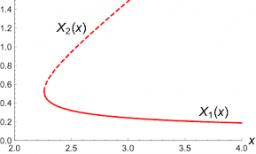# Expression 4451

Find the largest natural number d that has that property for any natural number
the number n is the value of the expression

V (n) = n ^ 4 + 11n2−12

is divisible by d.

d =  12

### Step-by-step explanation:Did you find an error or inaccuracy? Feel free to write us. Thank you!

Tips for related online calculators
Are you looking for help with calculating roots of a quadratic equation?
Do you have a linear equation or system of equations and looking for its solution? Or do you have a quadratic equation?
Do you want to convert time units like minutes to seconds?